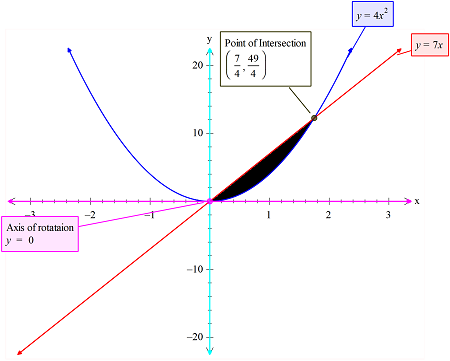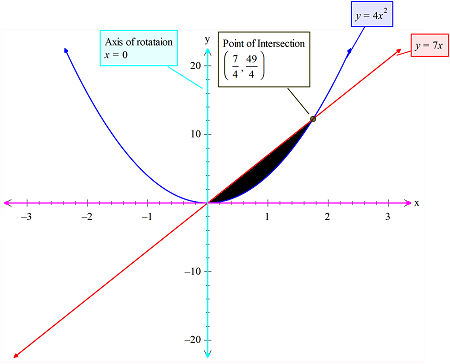# The region bounded by { y=4x^2 } and y=7x is to be routed about both axes and the volume...

## Question:

The region bounded by {eq}y=4x^2 {/eq} and y=7x is to be routed about both axes and the volume generated calculate by both the washer and the shell methods

A) when rotated about the x-axis is? (round to the nearest tenth)

B. when rotated about the y-axis is? (round to the nearest tenth)

## Definite Integrals:

The definite integral that is used in order to set up the volume integrals, will use either or both of the two methods. Those methods are the disk method or the washer method and the shell method or the cylindrical shell method. The integrals can be used to find the area too.

The region that is common betwen the curves equations is shown in black color in the figure below:1)

Here when the axis of rotation is the x-axis then the washer method will be used,

The inner and the outer radius is the top and the bottom curve as shown in the figure.

Now the volume is as per the integral set up :

{eq}V=\int_{0}^{\frac{7}{4}}\pi (7x)^2-(4x^2)^2 dx\\ =\int _0^{\frac{7}{4}}49\pi x^2dx-\int _0^{\frac{7}{4}}16x^4dx\\ =49\pi \left[\frac{x^3}{3}\right]^{\frac{7}{4}}_0 -16\left[\frac{x^5}{5}\right]^{\frac{7}{4}}_0 \\ =\frac{16807\pi }{192}-\frac{16807}{320}\\ =222.48 {/eq}

2)

In the second case the curve and the axis is shown:Now when the axis of rotation is ay-axis, then the shell method is to be used.

The radius of the shell is:

{eq}r= x\\ {/eq}

and the height is :

{eq}h=(7x-4x^2) {/eq}

So now the volume integral set up is as given by the expression:

{eq}V=\int_{0}^{\frac{7}{4}}2\pi x (7x-4x^2)dx\\ =2\pi \left[\frac{1}{2}\left(x^2\left(7x-4x^2\right)-2\cdot \int \frac{x^2\left(7-8x\right)}{2}dx\right)\right]^{\frac{7}{4}}_0\\ =2\pi \left[\frac{1}{2}\left(x^2\left(7x-4x^2\right)-2\left(\frac{7x^3}{6}-x^4\right)\right)\right]^{\frac{7}{4}}_0\\ =2\pi \left[\frac{1}{3}x^3\left(-3x+7\right)\right]^{\frac{7}{4}}_0\\ =\frac{2401\pi }{384} \\ =19.64 {/eq}